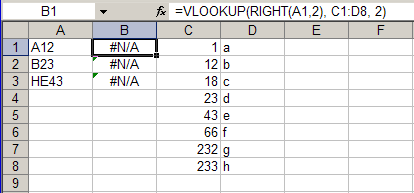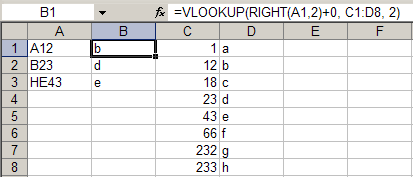## 2007.11.21 09:52 PM

### Excel Text-to-Numbers: Method 8

There's a Microsoft Knowledge Base article that describes a number of different ways to convert text representing numbers into actual numbers in Excel. The KB article outlines a number of methods for doing this, where the applicability of each method depends on, among other things, how the data is sourced and how much data you're faced with:

• Method 1: Use the Error Button
• Method 2: Retype the Values in the Cells
• Method 3: Edit Directly in the Cell
• Method 4: Use the Paste Special Command
• Method 5: Remove Hidden Characters and Spaces
• Method 6: Use a Visual Basic for Applications Procedure
• Method 7: Use the Text to Columns Command

I recently ran into a workbook whose particular number-as-text issue did not lend itself to any of these solutions directly. In this case, the rather large and complex workbook included numerous formulas that were, mid-formula, parsing the numerical portions out of some text values and then using them as the source for lookups in a range of numbers. The problem was that the parsed-out and concatenated number portions resulted in text values, not numbers, so they weren't being found in the range of numbers. Below is a screen-shot of a simplified example:Notice how the RIGHT() function, a simple stand-in for the actual formulas' parsing and concatenation logic, results in a text value, even though the text represents a numerical value, and so isn't found with VLOOKUP() in the range of numbers.

Reviewing my options, it seemed Method 5 was the most likely solution. Contrary to its name, Method 5 doesn't actually suggest that you manually clean up the data by removing hidden characters and spaces, which is an obvious solution for some types of data issues, but instead describes how to use a formula to convert unclean textual representations of numbers into clean numeric values. The formula it provides by way of example is:

```=VALUE(TRIM(CLEAN(A1)))
```

For my purposes, just the VALUE() function would suffice, but this presented a problem for this particular workbook and its troubled formulas. The problem was that the formulas were too long. Well, not too long yet, but the addition of seven more characters pushed a number of them over the 1,024 character limit. I might have mentioned that this was a terribly, and perhaps needlessly, complex workbook. And, unfortunately, it was not my workbook, so doing recon on some uncharted territory elsewhere in the workbook to lay down just the number parsing, concatenating, and now VALUE() converting, portions of the formulas was not an option.

The solution, which is the point of this post, was to eschew the VALUE() function and simply add 0 to the parsed values. Turns out that if you've got text that represents a valid number, adding 0 to it will convert it into an actual number. Try it yourself:

```="1"+0
```

See? A number.

Below is a screen-shot of the same sample from above, but this time with the addition of 0 to the text-returning RIGHT()-parsed number values: